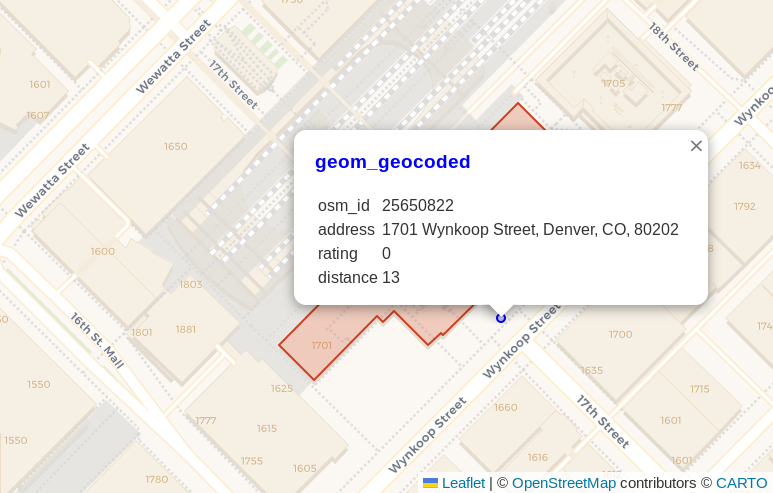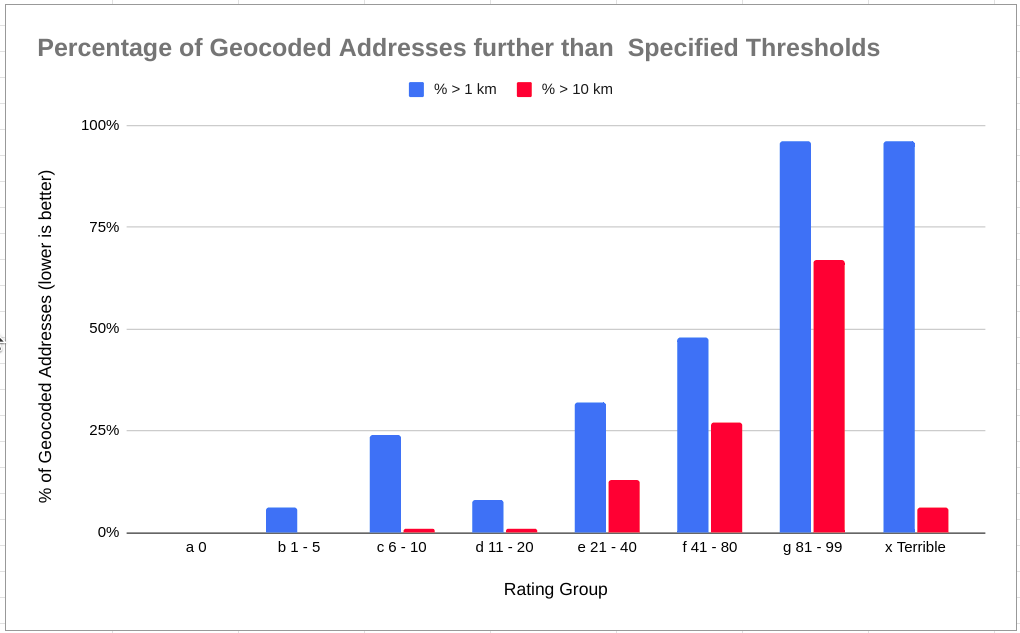My book Mastering PostGIS and OpenStreetMap is available!

# Geocode Addresses with PostGIS and Tiger/LINE

By Ryan Lambert -- Published October 10, 2023

My previous post, Setup Geocoder with PostGIS and Tiger/LINE, prepared a PostGIS geocoder with TIGER/Line data for Colorado. This post uses that setup to bulk geocode addresses from OpenStreetMap buildings to try to determine the accuracy of the geometry data derived from the input addresses.

## Quality Expectations

Let's get this out of the way: No geocoding process is going to be perfectly accurate.

There are a variety of contributing factors to the data quality. The geocoder data source is pretty decent, coming from the U.S. Census Bureau's TIGER/Line data set. The vintage is 2022, and this is as recent as I can load today using this data source. We should to understand that this will not contain any new development or changes from the past year (or so).

The OpenStreetMap data is also a source of error. It is certain that there are typos, outdated addresses, and other inaccuracies from the OpenStreetMap data. Some degree of that has to be expected with nearly any data source. The region of OpenStreetMap used will also be an influence, some regions just don't have many boots-on-the-ground editing and validating OpenStreetMap data.

Another factor with the way I check accuracy in this post is that the geocoder will point to a likely street address which is near to the physical street. Many buildings are set back a good distance from the road its address is associated with. The screenshot below is from my prior post and showed the geocoded location for Union Station in Denver was roughly a dozen meters away from the front entrance of the building itself.With this example in mind, I'm setting my general expectation of quality data to be within 250 meters. This gives leeway for houses with long driveways, new developments, and some basic generalization for areas that are downright tricky. I'll consider accuracy within 1 kilometer to be decent and locations off more than 10 kilometers to be poor quality. The 1 - 10 km will be that gray area we'll call the "Meh, maybe?" range.

Remember, some of the larger distance errors may be simple typos in the OpenStreetMap data. Colorado is filled with street name series such as Elm Street, Elm Way, Elm Court, Elm Place, Elm Boulevard and Elm Circle.

## OpenStreetMap Buildings

I loaded Colorado buildings from OpenStreetMap using PgOSM Flex as described in my post Load the Right Amount of OpenStreetMap Data. The following query shows there are 218,040 buildings with addresses including housenumber, street, city, and state. This gives us the addresses with the best chance of being successfully geocoded.

``````SELECT COUNT(*)
FROM osm_co_building.building_polygon
WHERE housenumber IS NOT NULL
AND street IS NOT NULL
AND city IS NOT NULL
AND state IS NOT NULL
;

┌────────┐
│ count  │
╞════════╡
│ 218040 │
└────────┘
``````

The following query creates a new table from the `geocode()` function's results. This query took 16 minutes on my laptop with the default `max_parallel_workers_per_gather = 2` set. It does run in parallel so setting that up to 4 or 6 likely would benefit the timing. This process also would likely run faster by using smaller batches (e.g. 100 - 100,000 at a time). This post, however, is not focused on performance so I went with the simple approach.

``````CREATE TABLE osm_co_building.building_polygon_geocoded_no_dups AS
gc.rating,
ST_Transform(gc.geomout, 3857) AS geom_geocoded,
b.geom
FROM osm_co_building.building_polygon b
LEFT JOIN LATERAL geocode(address, 1) gc ON True
WHERE housenumber IS NOT NULL
AND street IS NOT NULL
AND city IS NOT NULL
AND state IS NOT NULL
;
``````

I want to report on the accuracy of the location, so I materialize the `ST_Distance()` calculation using a generated column. The geometries are transformed to SRID 2773 to provide more accurate calculations for Colorado.

``````ALTER TABLE osm_co_building.building_polygon_geocoded_no_dups
GENERATED ALWAYS AS (ST_Distance(ST_Transform(geom, 2773), ST_Transform(geom_geocoded, 2773))) STORED
;
``````

The next query creates the `geocoded_with_ranks` table with rating and distance groupings for aggregations in the following sections.

``````DROP TABLE IF EXISTS geocoded_with_ranks;
CREATE TABLE geocoded_with_ranks AS
CASE WHEN rating = 0 THEN 'a 0'
WHEN rating > 0 AND rating <= 5 THEN 'b 1 - 5'
WHEN rating > 5 AND rating <= 10 THEN 'c 6 - 10'
WHEN rating > 10 AND rating <= 20 THEN 'd 11 - 20'
WHEN rating > 20 AND rating <= 40 THEN 'e 21 - 40'
WHEN rating > 40 AND rating <= 80 THEN 'f 41 - 80'
WHEN rating > 80 AND rating <= 99 THEN 'g 81 - 99'
WHEN rating = 100 THEN 'x Terrible'
ELSE 'z Not Geocoded'
END AS rating_group,
distance_from_original,
CASE WHEN distance_from_original < 250 THEN 'a < 250 meters'
WHEN distance_from_original < 1000 THEN 'b < 1km'
WHEN distance_from_original < 10000 THEN 'c < 10km'
ELSE 'z > 10km'
END AS distance_group,
geom_geocoded, geom
FROM osm_co_building.building_polygon_geocoded_no_dups
;
``````

Of the 218,040 input addresses, only 81 (0.04%) were not geocoded at all.

``````SELECT COUNT(*)
FROM geocoded_with_ranks
WHERE rating_group LIKE 'z%'
;
``````

## Accuracy compared to OpenStreetMap

The first approach to examining accuracy was comparing the geometry generated by geocoding to the polygon from the OpenStreetMap data. The following query shows that 91.5% of geocoded points were with 250 meters of their source polygon. I'm pretty happy with that number! Additionally, another 4.2% of addresses were between 250 meters and 1 kilometer from the OSM source. This means that 96% of geocoded addresses were within 1 km or less.

``````SELECT distance_group,
COUNT(*) AS cnt,
AVG(rating)::INT AS avg_rating,
AVG(distance_from_original)::BIGINT AS avg_geocode_distance,
MAX(distance_from_original)::BIGINT AS max_geocode_distance
FROM geocoded_with_ranks
GROUP BY 1
ORDER BY 1
;

┌────────────────┬────────┬────────────┬──────────────────────┬──────────────────────┐
│ distance_group │  cnt   │ avg_rating │ avg_geocode_distance │ max_geocode_distance │
╞════════════════╪════════╪════════════╪══════════════════════╪══════════════════════╡
│ a < 250 meters │ 199526 │          2 │                   34 │                  250 │
│ b < 1km        │   9117 │          7 │                  481 │                 1000 │
│ c < 10km       │   7556 │         28 │                 3677 │                 9999 │
│ z > 10km       │   1841 │         52 │               122521 │               556278 │
└────────────────┴────────┴────────────┴──────────────────────┴──────────────────────┘
``````

For the work I do, that's great! The most common way I use data that has been geocoded is to join it to an area, such as H3 hexagons at resolution 7. Errors in placement under 1km are typically too small to make a significant impact on these types of analysis.

## Accuracy by Rating

Having verified that the vast majority of geocoded points are accurate within 250 meters, how can we gauge this accuracy in more real-world solutions where we're geocoding for a reason? The `geocode()` results include a `rating` This section looks at how the `rating` values align with the accuracy against the OpenStreetMap data.

The following query aggregates geocoded addresses to their rating group. It calculates what percentage of results were further than 1 kilometer and what percentage were further than 10 kilometers from the source OpenStreetMap data. The data is visualized in the chart following the query.

``````SELECT rating_group, COUNT(*) AS cnt_total,
((COUNT(*) FILTER (WHERE distance_from_original > 1000))::NUMERIC
/ COUNT(*))::NUMERIC(3,2)
AS per_gt_1km,
((COUNT(*) FILTER (WHERE distance_from_original > 10000))::NUMERIC
/ COUNT(*))::NUMERIC(3,2)
AS per_gt_10km
FROM geocoded_with_ranks
WHERE rating_group NOT LIKE 'z%'
GROUP BY 1
ORDER BY 1
;

┌────────────────┬───────────┬────────────┬─────────────┐
│  rating_group  │ cnt_total │ per_gt_1km │ per_gt_10km │
╞════════════════╪═══════════╪════════════╪═════════════╡
│ a 0            │    162105 │       0.00 │        0.00 │
│ b 1 - 5        │     20628 │       0.06 │        0.00 │
│ c 6 - 10       │     10068 │       0.24 │        0.01 │
│ d 11 - 20      │     17242 │       0.08 │        0.01 │
│ e 21 - 40      │      3867 │       0.32 │        0.13 │
│ f 41 - 80      │      2462 │       0.48 │        0.27 │
│ g 81 - 99      │       406 │       0.96 │        0.67 │
│ x Terrible     │      1181 │       0.96 │        0.06 │
└────────────────┴───────────┴────────────┴─────────────┘
``````The above chart gives me a good impression about the records with a geocode ranking of 20 and lower. The following query looks at that group's accuracy. In summary, 93% of geocoded addresses are within 250 meters, 97% within 1 kilometer, and 99.9% within 10 kilometers. For most regional work, this is pretty darn good. For automated delivery using drones... well, you'll want to ensure end users verify and/or adjust their location. :)

WARNING - I flipped the logic here, now looking at less than instead of greater than.

``````SELECT COUNT(*) AS cnt_total,
((COUNT(*) FILTER (WHERE distance_from_original <= 250))::NUMERIC
/ COUNT(*))::NUMERIC(4,3)
AS per_lt_250m,
((COUNT(*) FILTER (WHERE distance_from_original <= 1000))::NUMERIC
/ COUNT(*))::NUMERIC(4,3)
AS per_lt_1km,
((COUNT(*) FILTER (WHERE distance_from_original <= 10000))::NUMERIC
/ COUNT(*))::NUMERIC(4,3)
AS per_lt_10km
FROM geocoded_with_ranks
WHERE rating <= 20
;

┌───────────┬─────────────┬────────────┬─────────────┐
│ cnt_total │ per_lt_250m │ per_lt_1km │ per_lt_10km │
╞═══════════╪═════════════╪════════════╪═════════════╡
│    210043 │       0.934 │      0.974 │       0.999 │
└───────────┴─────────────┴────────────┴─────────────┘
``````

## Summary

This post showed that the PostGIS Tiger/LINE geocoder created in my prior post provides accuracy good enough for the types of projects I use it for. When filtering for `rating <= 20` I can expect that 97% of the geocoded points are within 1 kilometer of reality. I use this type of data for generalized spatial trends aggregated to larger polygons, so this level of drift is negligible. Omitting the filter on `rating` is even an option if the tolerance for issues is allowed to be a little wider.